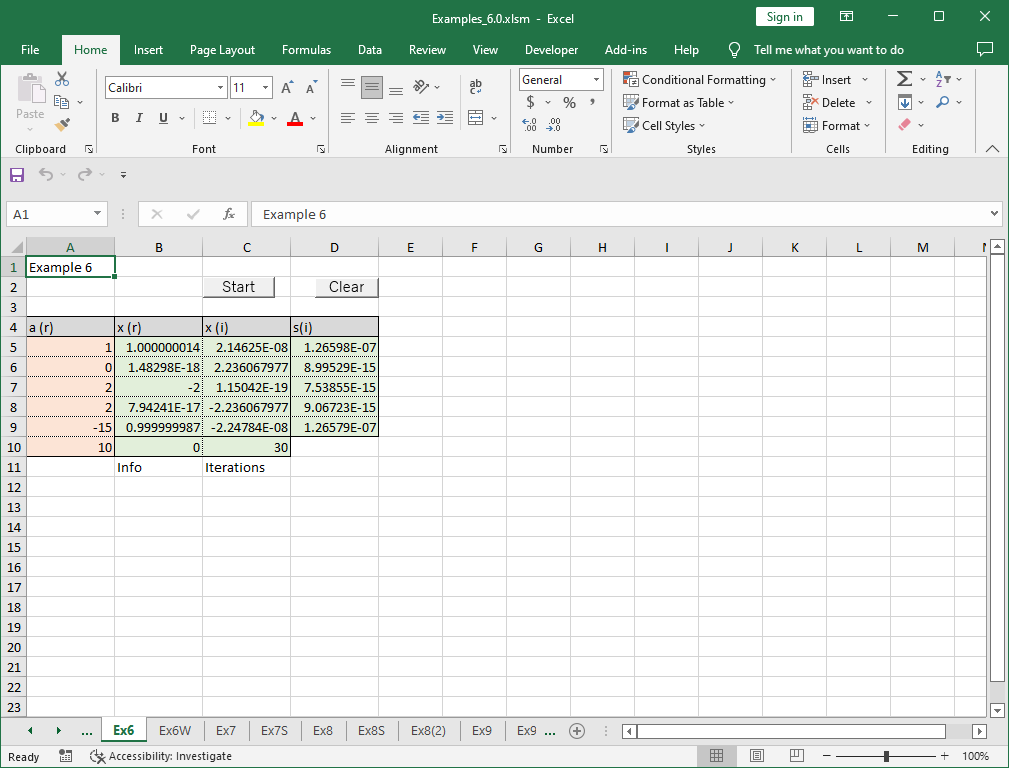# 6. Algebraic equations (roots of polynomials)

Note – The example worksheets are available for download. See 1. How to obtain and use example worksheets.

Examples of solving algebraic equations (finding roots of polynomials) by XLPack are shown below.

### Example

Now, we solve the following equation.

```
x5 + 2x3 + 2x2 - 15x + 10 = 0

```

This can be factorized as follows.

```
(x - 1)2(x + 2)(x2 + 5) = 0

```

Then, the solutions are 1(multiple root), -2, and ±√5i.

Two solving methods using worksheet function WRpzero2 and VBA subroutine Rpzero2 are described.

### Solving problem with worksheet function

Enter the data of coefficients ai of the equation into the appropriate cells (orange cells) in the worksheet, and select (n + 1) x 3 cells where the solution (x(r) and x(i)) and the error bounds (s(i)) are to be placeed (green cells) and enter woeksheet function WRpzero2.

The parameters required by WRpzero2 are N and A. N is the order of the equations (e.g. 3), A is the cell range of the coefficients of the equation. Now let us denote the equation a0 xn + a1 xn-1 + … + an-1 x + an = 0, the coefficients are represented by the real numbers a0, a1, … , an.Then press Ctrl + Shift + Enter.Now, we’ve got the five solutions correctly. The most right column (s(i)) shows the error bounds. The bottom line shows, from the left, the return code and the number of iterations required for convergence.

For the multiple root (1), the accuracy is dropped to about half of those for other roots. It should be noted that, in solving algebraic equations, the effective number of digits of the computed m multiple root value becomes about 1/m of that of single root.

### Solving problem with VBA program

Let’s solve the same example problem by VBA program. The following is an example code using VBA library subroutine Rpzero2.

``````Sub Start()
Const NMax = 10
Dim N As Long
Dim A(NMax) As Double, XR(NMax) As Double, XI(NMax) As Double, S(NMax) As Double
Dim IFlag As Long, Info As Long, Iter As Long, I As Long
'--- Input data
N = 5
For I = 0 To N
A(I) = Cells(5 + I, 1)
Next
'--- Compute roots of equation
IFlag = 0
Call Rpzero2(N, A(), XR(), XI(), IFlag, S(), Info, Iter)
Cells(10, 2) = Info
Cells(10, 3) = Iter
'--- Output roots
For I = 0 To N - 1
Cells(5 + I, 2) = XR(I)
Cells(5 + I, 3) = XI(I)
Cells(5 + I, 4) = S(I)
Next
End Sub``````

After entering the data of coefficients into the appropriate cells (orange cells), the following result was obtained by running the macro “Start”. Unlike the example using worksheet functions, you cannot obtain results by simply entering the data, and you need to run a VBA program.